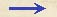Discorsi Propositions 1/02-th-02 Discorsi Proposition1/02-th-02{10} THEOREMA II, PROPOSITIO II. THEOREM II, PROPOSITION II Si mobile temporibus aequalibus duo pertranseat spatia, erunt ipsa spatia inter se ut velocitates. If a moving particle traverses two distances in equal intervals of time, these distances will bear to each other the same ratio as the speeds. Et si spatia sint ut velocitates, tempora erunt aequalia. And conversely if the distances are as the speeds then the times are equal. Assumpta enim superiori figura, sint duo spatia AB, BC transacta aequalibus temporibus, spatium quidem AB cum velocitate DE, et spatium BC cum velocitate EF: dico, spatium AB ad spatium BC esse ut DE velocitas ad velocitatem EF. Sumptis enim utrinque, ut supra, et spatiorum et velocitatum aeque multiplicibus secundum quamcunque multiplicationem, scilicet GB et IE ipsorum AB et DE, pariterque HB, KE ipsorum BC, EF, {20} concludetur, eodem modo ut supra, multiplicia GB, IE vel una deficere, vel aequari, vel excedere, aeque multiplicia BH, EK.Igitur et manifestum est propositum. Referring to Fig. 40, let AB and BC represent the two distances traversed in equal time-intervals, the distance AB for instance with the velocity DE, and the distance BC with the velocity EF. Then, I say, the distance AB is to the distance BC as the velocity DE is to the velocity EF. For if equal multiples of both distances and speeds be taken, as above, namely, GB and IE of AB and DE respectively, and in like manner HB and KE of BC and EF, then one may infer, in the same manner as above, that the multiples GB and IE are either less than, equal to, or greater than equal multiples of BH and EK. Hence the theorem is established.Discorsi Propositions 1/02-th-02 Discorsi Proposition1/02-th-02